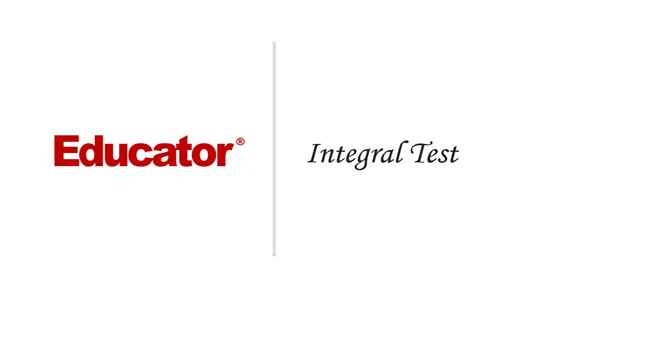Professor Murray

Integral Test

Slide Duration:

Integration by Parts

24m 52s

Intro
0:00
Important Equation
0:07
Where It Comes From (Product Rule)
0:20
Why Use It?
0:35
Lecture Example 1
1:24
Lecture Example 2
3:30
Shortcut: Tabular Integration
7:34
Example
7:52
Lecture Example 3
10:00
Mnemonic: LIATE
14:44
Ln, Inverse, Algebra, Trigonometry, e
15:38
-1
-2
Integration of Trigonometric Functions

25m 30s

Intro
0:00
Important Equation
0:07
Powers (Odd and Even)
0:19
What To Do
1:03
Lecture Example 1
1:37
Lecture Example 2
3:12
Half-Angle Formulas
6:16
Both Powers Even
6:31
Lecture Example 3
7:06
Lecture Example 4
10:59
-1
-2
Trigonometric Substitutions

30m 9s

Intro
0:00
Important Equations
0:06
How They Work
0:35
Example
1:45
Remember: du and dx
2:50
Lecture Example 1
3:43
Lecture Example 2
10:01
Lecture Example 3
12:04
-1
-2
Partial Fractions

41m 22s

Intro
0:00
Overview
0:07
Why Use It?
0:18
Lecture Example 1
1:21
Lecture Example 2
6:52
Lecture Example 3
13:28
-1
-2
Integration Tables

20m

Intro
0:00
Using Tables
0:09
Match Exactly
0:32
Lecture Example 1
1:16
Lecture Example 2
5:28
Lecture Example 3
8:51
-1
-2
Trapezoidal Rule, Midpoint Rule, Left/Right Endpoint Rule

22m 36s

Intro
0:00
Trapezoidal Rule
0:13
Graphical Representation
0:20
How They Work
1:08
Formula
1:47
Why a Trapezoid?
2:53
Lecture Example 1
5:10
Midpoint Rule
8:23
Why Midpoints?
8:56
Formula
9:37
Lecture Example 2
11:22
Left/Right Endpoint Rule
13:54
Left Endpoint
14:08
Right Endpoint
14:39
Lecture Example 3
15:32
-1
-2
Simpson's Rule

21m 8s

Intro
0:00
Important Equation
0:03
Estimating Area
0:28
Difference from Previous Methods
0:50
General Principle
1:09
Lecture Example 1
3:49
Lecture Example 2
6:32
Lecture Example 3
9:07
-1
-2
Improper Integration

44m 18s

Intro
0:00
Horizontal and Vertical Asymptotes
0:04
Example: Horizontal
0:16
Formal Notation
0:37
Example: Vertical
1:58
Formal Notation
2:29
Lecture Example 1
5:01
Lecture Example 2
7:41
Lecture Example 3
11:32
Lecture Example 4
15:49
Formulas to Remember
18:26
Improper Integrals
18:36
Lecture Example 5
21:34
Lecture Example 6 (Hidden Discontinuities)
26:51
-1
-2
Section 2: Applications of Integrals, part 2
Arclength

23m 20s

Intro
0:00
Important Equation
0:04
Why It Works
0:49
Common Mistake
1:21
Lecture Example 1
2:14
Lecture Example 2
6:26
Lecture Example 3
10:49
-1
-2
Surface Area of Revolution

28m 53s

Intro
0:00
Important Equation
0:05
Surface Area
0:38
Relation to Arclength
1:11
Lecture Example 1
1:46
Lecture Example 2
4:29
Lecture Example 3
9:34
-1
-2
Hydrostatic Pressure

24m 37s

Intro
0:00
Important Equation
0:09
Main Idea
0:12
Different Forces
0:45
Weight Density Constant
1:10
Variables (Depth and Width)
2:21
Lecture Example 1
3:28
-1
-2
Center of Mass

25m 39s

Intro
0:00
Important Equation
0:07
Main Idea
0:25
Centroid
1:00
Area
1:28
Lecture Example 1
1:44
Lecture Example 2
6:13
Lecture Example 3
10:04
-1
-2
Section 3: Parametric Functions
Parametric Curves

22m 26s

Intro
0:00
Important Equations
0:05
Slope of Tangent Line
0:30
Arc length
1:03
Lecture Example 1
1:40
Lecture Example 2
4:23
Lecture Example 3
8:38
-1
-2
Polar Coordinates

30m 59s

Intro
0:00
Important Equations
0:05
Polar Coordinates in Calculus
0:42
Area
0:58
Arc length
1:41
Lecture Example 1
2:14
Lecture Example 2
4:12
Lecture Example 3
10:06
-1
-2
Section 4: Sequences and Series
Sequences

31m 13s

Intro
0:00
Definition and Theorem
0:05
Monotonically Increasing
0:25
Monotonically Decreasing
0:40
Monotonic
0:48
Bounded
1:00
Theorem
1:11
Lecture Example 1
1:31
Lecture Example 2
11:06
Lecture Example 3
14:03
-1
-2
Series

31m 46s

Intro
0:00
Important Definitions
0:05
Sigma Notation
0:13
Sequence of Partial Sums
0:30
Converging to a Limit
1:49
Diverging to Infinite
2:20
Geometric Series
2:40
Common Ratio
2:47
Sum of a Geometric Series
3:09
Test for Divergence
5:11
Not for Convergence
6:06
Lecture Example 1
8:32
Lecture Example 2
10:25
Lecture Example 3
16:26
-1
-2
Integral Test

23m 26s

Intro
0:00
Important Theorem and Definition
0:05
Three Conditions
0:25
Converging and Diverging
0:51
P-Series
1:11
Lecture Example 1
2:19
Lecture Example 2
5:08
Lecture Example 3
6:38
-1
-2
Comparison Test

22m 44s

Intro
0:00
Important Tests
0:01
Comparison Test
0:22
Limit Comparison Test
1:05
Lecture Example 1
1:44
Lecture Example 2
3:52
Lecture Example 3
6:01
Lecture Example 4
10:04
-1
-2
Alternating Series

25m 26s

Intro
0:00
Main Theorems
0:05
Alternation Series Test (Leibniz)
0:11
How It Works
0:26
Two Conditions
0:46
Never Use for Divergence
1:12
Estimates of Sums
1:50
Lecture Example 1
3:19
Lecture Example 2
4:46
Lecture Example 3
6:28
-1
-2
Ratio Test and Root Test

33m 27s

Intro
0:00
Theorems and Definitions
0:06
Two Common Questions
0:17
Absolutely Convergent
0:45
Conditionally Convergent
1:18
Divergent
1:51
Missing Case
2:02
Ratio Test
3:07
Root Test
4:45
Lecture Example 1
5:46
Lecture Example 2
9:23
Lecture Example 3
13:13
-1
-2
Power Series

38m 36s

Intro
0:00
Main Definitions and Pattern
0:07
What Is The Point
0:22
0:45
Interval of Convergence
2:42
Lecture Example 1
3:24
Lecture Example 2
10:55
Lecture Example 3
14:44
-1
-2
Section 5: Taylor and Maclaurin Series
Taylor Series and Maclaurin Series

30m 18s

Intro
0:00
Taylor and Maclaurin Series
0:08
Taylor Series
0:12
Maclaurin Series
0:59
Taylor Polynomial
1:20
Lecture Example 1
2:35
Lecture Example 2
6:51
Lecture Example 3
11:38
Lecture Example 4
17:29
-1
-2
Taylor Polynomial Applications

50m 50s

Intro
0:00
Main Formulas
0:06
Alternating Series Error Bound
0:28
Taylor's Remainder Theorem
1:18
Lecture Example 1
3:09
Lecture Example 2
9:08
Lecture Example 3
17:35
-1
-2
Bookmark & Share Embed

## Copy & Paste this embed code into your website’s HTML

Please ensure that your website editor is in text mode when you paste the code.
(In Wordpress, the mode button is on the top right corner.)
×
• - Allow users to view the embedded video in full-size.
Since this lesson is not free, only the preview will appear on your website.

• ## Related Books1 answer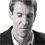Last reply by: Dr. William MurrayMon Oct 21, 2013 10:31 PMPost by Wael Saleh on October 20, 2013Great job, As you know there are many types of tests, Therefore, I have problem !!  Are there any certain signals in question describe which kind of tests should I use it to solve problem?Best regards,1 answerLast reply by: Dr. William MurraySun Apr 28, 2013 11:12 AMPost by Megan Kell on April 10, 2012I'm so confused... I understand the p-series method and the integral method, but what do they have to do to each other? and at the beginning of this lecture, he talks about the three points you must identify before doing the integral test, but yet he only covers that in additional example IV, although he should have demonstrated this in Example 3 as well..? The thought process in this lecture is not very clearly executed.1 answerLast reply by: Dr. William MurraySun Apr 28, 2013 11:04 AMPost by Jaspreet Singh on March 15, 2010There's a typo in the notes, for number 3 it should be "Is f'(x) negative"1 answerLast reply by: Dr. William MurraySun Apr 28, 2013 10:44 AMPost by John Haddad on February 10, 2010Tabular integration is a great technique for Ex 3, unfortunately my university disallows it's use on exams; but it'll come in handy for multiple choice problems.

### Integral Test

Main theorem and definition:

Suppose f (x) is a function and you want to know if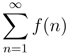converges.

Check three conditions:

1. Is f (x) continuous?

2. Is f (x) always positive?

3. Is f (x) decreasing? (i.e., is f (x) negative?)

Then if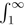f (x)dx converges, thenconverges.

And iff (x)dx diverges, thendiverges.

Definition: A p-series is a series of the form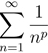. Using the Integral Test, we can show that the series converges if p> 1 and diverges to infinity if p ≤ 1.

Hints and tips:

• It’s important to remember that the Integral Test only applies to series with positive terms. However, after you learn about absolute convergence later, you may be able to use it for series with some negative terms by taking their absolute value and seeing if they are absolutely convergent.

• The Integral Test is one of the few two-way tests − you can use it to conclude that a series converges or that it diverges.

• Don’t make the common mistake of confusing p-series with geometric series. p-series has the n in the base and a constant exponent. Geometric series has a constant base and n in the exponent. When a geoemetric series converges, we have formulas to tell us what it converges to. When a p-series converges, we usually don’t know what it converges to.

• It’s worth memorizing the rule for p-series, since these are often used later in conjunction with the Comparison Test.

• Remember that the Integral Test never tells you exactly what a series converges to.

### Integral Test

Can the Integral Test be applied on the ∑n = 1 [1/(n − 1)] ?
Analyze conditions
If f(n) = [1/(n − 1)], then f(x) is not continuous on the intertval [1,∞] on x = 1 Thus the Integral Test cannot be applied
Can the Integral Test be applied on the ∑n = 1 [(n3)/(n + 1)] ?
Analyze conditions
If f(n) = [(n3)/(n + 1)], then f(1) < f(2) and thus f(x) is increasing.
Thus the Integral Test cannot be applied.
Solve ∫ [(x3)/([(x4)/4] + 1)]dx
• Use substitution with u = [(x4)/4] + 1
• u = [(x4)/4] + 1
• du = x3dx
Integrate with substitution
[(x3)/([(x4)/4] + 1)]dx = ∫ [du/u] = ln|[(x4)/4] + 1| + C
Does the series ∑[(n3)/([(n4)/4] + 1)] converge?
• Integrate f(x) from 1 to ∞
• 1 [(n3)/([(n4)/4] + 1)] = ∫1 [(x3)/([(x4)/4] + 1)]
• Integrate the improper integral
• 1 [(x3)/([(x4)/4] + 1)] = limk∞1k [(x3)/([(x4)/4] + 1)]
• = limk∞ [ ln|[(x4)/4] + 1| ]1k
• = limk∞ [ ln|[(k4)/4] + 1| − ln|[1/4] + 1| ]
• = ∞
Thus the series diverges
Solve ∫ [(x + 61)/(ex)]dx
• Setup integration by parts
• u = x + 61
• du = dx
• dv = [1/(ex)]
• v = − [1/(ex)]
[(x + 61)/(ex)]dx = − [(x + 61)/(ex)] + ∫ [dx/(ex)] = − [(x + 61)/(ex)] − [1/(ex)] + C = [(x + 60)/(ex)] + C
Does the series ∑[(x + 61)/(ex)] converge or diverge?
• Integrate f(x) from 1 to ∞
• 1 [(n3)/([(n4)/4] + 1)] = ∫1 [(x + 61)/(ex)]
• Integrate the improper integral
• 1 [(x + 61)/(ex)] = limk∞1k [(x + 61)/(ex)]
• = limk∞ [ [(x + 60)/(ex)] ]1k
• = limk∞ [ [(k + 60)/(ex)] − [61/(e)] ]
• = limk∞ [ [k/(ex)] − [1/e] ]
• = 0 − [1/e]
• = − [1/e]
Thus the series converges.
Solve ∫ [1/(x3)]dx
Integrate
[1/(x3)]dx = − [(x − 2)/2] + C
Does the series 7 + [7/8] + [7/27] + [7/64] + ... converge or diverge?
• Determine the series formula
• 7 + [7/8] + [7/27] + [7/64] + ... = ∑[7/(n3)]
• Integrate f(x) from 1 to ∞
• 1 [7/(n3)] = ∫1 [7/(x3)]
• = 7∫1 [1/(x3)]
• = 7limk∞ [ − [1/(2x2)] ]1k
• = 7limk∞ [ − [1/(2k2)] + [1/2] ]
• = [7/2]
Thus the series converges
Determine if the series ∑n = 1 [1/(3√{n})] converges with P - series conditions
Analyze pp = [1/3] ≤ 1, thus the series diverges
Determine if the series ∑n = 1 [1/(3√{n})] converges with P - series conditions
• Integrate f(x) from 1 to ∞
• 1 [1/(3√{n})] = ∫1 [1/(3√{x})]
• = limk∞ [ [3/2]x[2/3] ]1
• = [3/2]limk∞ [ k[2/3] − 1 ]
• = ∞
Thus the series diverges.

*These practice questions are only helpful when you work on them offline on a piece of paper and then use the solution steps function to check your answer.

### Integral Test

Lecture Slides are screen-captured images of important points in the lecture. Students can download and print out these lecture slide images to do practice problems as well as take notes while watching the lecture.

• Intro 0:00
• Important Theorem and Definition 0:05
• Three Conditions
• Converging and Diverging
• P-Series
• Lecture Example 1 2:19
• Lecture Example 2 5:08
• Lecture Example 3 6:38

### Transcription: Integral Test

Hi, this is educator.com.0000

We are trying a couple more examples of the integral test.0002

The first one here is the summation from n=2 to infinity of 1/n × ln(n).0007

You are probably used to seeing series that start at n=1.0013

Here I had to start it at n=2.0018

If you plug it n=1, to the natural log, ln(1) = 0.0021

If we started at n=1, we would get 1/0 and that would not make sense.0026

That is why I had to start at n=2.0030

So the integral test says you look at what you are given for a series and you convert it into a function.0032

f(x) = 1/x ln(x).0037

There are three things you have to check.0047

One, whether it is continuous.0050

This is a continuous function, because we are only looking at values of x bigger than 2.0056

There is no question about dividing by 0 in there.0062

Whether it is positive and this is positive as long as, well x is always positive.0066

This is positive as long as ln(x) is greater than 0, which it is,0079

Since we are only looking at values of x bigger than 2.0083

The third question is whether the function is decreasing.0093

To check that, we have to look a the derivative f'(x).0098

The derivative of that, if we think of f(x) as x × ln(x)-1.0105

The derivative is -x log(x)-2 × the derivative of x log(x).0112

That is a product so we have to use the product rule.0125

That is x × the derivative of log(x) + log(x) times derivative of x,0126

The derivative of x is just 1.0136

This simplifies down into -1 + log(x)/x2 × log(x)2.0137

If we look at that, 1 + log(x) > 0.0151

x2 × log(x) 2 > 0.0157

We have got numerator, denominator positive, but then we have got a big negative sign on the outside.0162

So, this whole thing is going to be negative.0169

Remember that was going to be the derivative, that means it has a negative derivative.0173

That means the function is decreasing.0179

Having checked those 3 conditions, it is ok to use the integral test.0183

We are going to look at the integral from 2 to infinity of 1/x log(x) dx.0193

That is not such a bad integral to do.0205

We can use u = ln(x).0208

Then, du = just 1/x dx.0211

We have got the integral from x=2 to x=infinity of 1/u du.0217

So, that is the integral of ln(u) evaluated from x = 2, well a value of t going to infinity.0230

That is the ln(ln(x)), because we have to change u back into the ln(x).0244

Again, evaluated from x=2 to x=a value that goes to infinity.0253

The second part of this is very easy.0261

- ln(ln(2)) is no problem there.0264

- ln(ln(2)) is just some number.0268

However, when we take ln(of some number going to infinity), that will go to infinity.0272

We actually want ln(that), but then we have ln(something going to infinity),0277

This whole thing diverges to infinity.0291

The integral diverges to infinity.0298

So, we can say the series 1/n log(n) diverges to infinity by the integral test.0302

Let us recap what happened there.0321

We were given the series of 1/n log(n).0330

We converted that into a function and checked these 3 conditions, continuous, positive, and decreasing.0335

The trickiest one there was decreasing.0340

We had to look at the derivative and work it through and check that it was negative.0343

Once we verified those 3 conditions, it is OK to use the integral test.0347

We look at the improper integral of 1/x log(x) from 2 to infinity.0353

That, we work out the calculus and that turns out to be ln(ln(x)).0358

Then when we plug in large values of x to ln(ln(x)), we get something that diverges to infinity,0365

So the integral test tells us that the original series diverges to infinity as well.0374

Let us try one more example of a problem that suggests the integral test.0000

Here we have the sum from n=1 to infinity of 1/n2 + 1.0005

We would set up f(x) = 1/n2 + 1.0012

Now, there are three conditions we need to check, continuous, positive, decreasing.0020

The continuous is pretty clear.0032

The positive is also pretty clear, no matter what x you put there that is going to be positive.0038

The decreasing, you should work out f'(x) and check that it is negative.0044

I am not going to work it out here, but it does turn out to be negative so it is ok to use the integral test.0049

Let us look at the integral from x=1 to x going to infinity of 1/x2+1 dx.0062

There are several ways to do that integral.0074

You can do it with a trig substitution, you would use x = tan(θ).0077

You could look it up in the table of integrals in the back of your calculus book.0081

Probably for this integral, it is one that you are going to see often enough that it is good to have the answer memorized.0087

The integral of 1/x2+1 = arctan(x).0096

That is one that if you have not memorized it yet,0103

You will probably see often enough as you are doing calculus, that it is worthwhile to memorize it.0104

Then we want to evaluate that from x=1 to a value that is going to infinity.0109

So, this is arctan(a number going to infinity) - arctan(1).0121

Now, remember what the arctangent function does.0132

It is the inverse of the tangent function.0136

There is the tangent function with asymptotes at -π/2 and π/2.0139

That is tan(x).0147

Arctan(x) says you flip that and so you get these horizontal asymptotes at -π/2 and π/2.0149

That is arctan(x).0163

When x goes to infinity, arctan(x) approaches π/2.0168

The limit there is π/2.0173

The arctan(1), well you think what angle as arctan(1) and if you remember your common values that is π/4.0177

So this improper integral converges to π/4.0187

What that tells us is that the series, 1/n2+1 converges by the integral test.0190

What it does not tell us is what it converges to.0211

Remember, the integral test does not tell you that.0221

I will remind you that we do not know from anything we have learned so far, what that series converges to.0225

Even though the integral came out to be π/4, that does not tell you anything about what the series converges to.0235

All we can say from the integral test is that the series converges.0244

To recap there, we were given a function, a series,0249

We convert it into a function,0255

We check ti see if it is continuous, positive and decreasing,0256

Decreasing is the challenging part, we have to check that its derivative is negative.0259

Once we verify those, we do the integral.0265

We find that the answer turns out to be a finite limit.0269

That does not turn out to be infinity.0274

That allows us to conclude that the series converges by the integral test.0278

But we do not know exactly what the series converges to.0284

This has been educator.com0286

Hi, this is educator.com and we are here to talk about the integral test.0000

The integral test is a way of determining when a series converges or diverges.0006

The way it often works is you will be given a series in this form.0013

f(n) is just some expression in terms of n.0017

What you do is convert that into a function of x.0020

Then there are 3 things you have to check before you can apply the integral test.0025

You have to check whether it is a continuous function,0029

You have to check whether it is always positive.0033

The integral test only works for series with positive terms.0036

You have to check whether it is decreasing.0040

In other words, is the derivative negative?0043

The derivative has to be negative to pass the integral test.0046

If you checked all three of those conditions, the integral test tells you that you can look at the integral of f(x) from 1 to infinity,0050

And if it converges, then your series converges.0060

If it diverges, then your series diverges.0064

We will try some examples of that in a moment.0069

In the meantime, let me give you 1 important definition that comes out of the integral test.0073

We are going to talk about p series.0077

That is a series of the form 1/np where p is a constant value.0080

P series lend themselves very nicely to integral tests.0088

Because, we figured out back in the integration section on improper integrals.0096

We figured out when the integral of dx/xp converges.0106

What we figured out was that the integral from 1 to infinity of dx/xp converges exactly when p > 1 and diverges to infinity if p < or = 1.0109

We will use that result to look at some series today.0127

You will use it also in your calculus homework.0131

Let us try out some examples of the integral test.0135

First one is to determine whether the series 1/sqrt(n) converges or diverges.0140

That one we can write it as 1/n1/2.0148

n = one to infinity.0157

That is now a p series.0162

With p = 1/2, 1/2 < or = to 1, so the series diverges to positive infinity.0169

Let me mention something about p series, because we will be seeing several examples of them.0198

We have also seen another kind of series called a geometric series.0204

These are frequently mixed up by students.0210

Let me mention what the difference is.0211

This n1/2 is a p series because the variable n is in the base, and the constant 1/2 is in the exponent, so that is a p series.0213

A geometric series, the general form of a p series is 1/np.0231

A geometric series, if you had something like 1/2n, where the constant is the base and the variable is in the exponent,0236

That is a geometric series.0250

We saw some examples of those earlier.0253

The general form of a geometric series would be the sum of rn,0258

Where r is the constant.0262

Remember in the p series, the exponent is the constant, and in the geometric series, the base is the constant.0269

Those two are very frequently mixed up by students.0277

They are different kinds of series and the rules for determining when they converge or diverge look similar on the surface.0280

You really have to remember separate rules for geometric rules and p series.0288

You have to be careful not to label a geometric series as a p series, or a p series as a geometric series.0297

This one is the series 1/n3/2.0309

Again, this is a p series, because the constant is in the exponent.0315

Here p is 3/2, which is bigger than 1.0324

So, the series converges.0332

Let me mention another feature of the integral test in a p series.0345

Which is that the integral test and the rules for when a p series converge,0351

None of those tell you what the series converges to.0357

We do not know from anything we have learned so far what the series converges to.0367

We do not know the limit of the series.0378

In that sense, the integral test and the p series rules are limited.0384

They will tell you whether the series converges or diverges, but if a series does converge, it will not tell you what it converges to.0389

Let us try another example here.0398

The sum of n2/en.0402

This one is a little more complicated and is not just a simple p series.0405

Let us look at f(x) = x2/xn.0409

We want to use the integral test to tell whether or not the series converges or diverges.0420

It will be easier to integrate this thing if we look at it as x2e-x.0424

I want to look at the improper integral from 1 to infinity of x2e-x dx.0431

That is a classic integral to solve by integration by parts.0444

I am going to use the trick of tabular integration that we learned in the integration by parts lecture to integrate this.0447

If you do not remember the tabular integration trick, you might want to go back and look at th integration by parts lecture.0460

I will also go through it slowly here.0468

If we take the derivatives of x2, we get 2x, 2 and then 0.0472

If we take the derivatives of e-x, the first integral is -e-x, the integral of that is just e-x again.0479

The integral of that is -e-x.0488

Then we write these diagonal lines and the signs +, -, +.0493

Then we multiply along the diagonal lines to get the answer x2e-x - 2xe-x -2e-x.0500

We want to evaluate that from x=1 to x=some value t which will go to infinity.0516

This tabular integration is a shorthand way of doing integration by parts.0527

You can also do integration by parts by using u and dv.0535

You should get the same answer here.0542

If you plug that in, e-x, this is the same as saying x2/e-x.0543

As we plug in infinite values there, larger and larger values of x,0551

The e-x dominates the x2.0560

When we take the limit as t goes to -infinity,0568

Since the e-x dominates the x2, we actually get 0 on each of these terms.0569

0-0-0, and now if we plug in x=1.0575

We get - a -, so that is a + 1/e + 2/e +2/e.0581

If you add those up to get 5/e, which is a finite number.0604

The conclusion we can draw from that is the series that we were given n2/en converges by the integral test.0613

If this integral had diverged to infinity, then we would have said the series diverges to infinity.0645

The integral test is nice in that it can give you either answer.0654

Unlike some of the other tests.0657

If this integral had diverged to infinity, we would have said the series diverged to infinity.0660

Where the integral test falls, and does not give us an answer, is it does not tell us exactly what the series converges to.0667

Even this 5/e is attempting to say, well that is the value of the interval, that must be the value of the series.0674

That is not safe.0682

This 5/e, we do not actually get that much from the numerical value 5/e.0684

The important thing is just that it is finite, and so it tells use the series converges.0689

We do not know using what we have learned so far what it converges to.0698

So, that is how the integral test works.0713

You take the series that you are given, convert it into a function, work out the integral from 1 to infinity of that function.0717

Then, if you get a finite answer, you can say the series converges.0727

If you get an infinite answer, you can say the series diverges.0733

We will try a couple more examples of that later on.0736

OR

### Start Learning Now

Our free lessons will get you started (Adobe Flash® required).